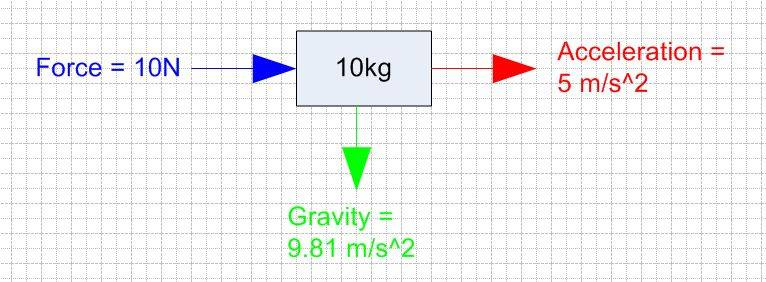# Understanding Newton's 2nd Law of Motion

Homework Statement:
Understanding Newton's 2nd Law of Motion
Relevant Equations:
NA
Hello all

I am trying to understanding Newton's 2md Law of Motion which states:-

Force = Mass * Acceleration

If I had a force of 10Newtons pushing an object along the ground (assume no friction) in a perfectly horizontal direction that has a Mass of 10kg then the objects acceleration would be 5m/s^2 in the horizontal direction.

My question why is gravity factored into this as shown in my sketch below:-Gravity is acting on the mass while a force of 10N is being applied - so my question is shouldn't gravity be considered as another force acting downwards which would effect the acceleration and the direction of the Mass?

I hope this makes sense?

Just to clarify, as is always done, ##F=\frac{dp}{dt}## where ##p=mv##. If you don't know about calculus, you get the equation you have because mass is usually taken as a constant.
As to your question, yes it should be and is considered as such, but not in the way you're thinking of it, probably. The object in your picture is moving horizontally, and since we're neglecting air resistance and assuming no friction, there's nothing besides the ##10N## force to affect its horizontal motion. Gravity is making the object accelerate downwards, but has no effect on its horizontal motion (since the force of friction is ##F=[(\mu=0)\times N]=0##).

Last edited:
ZapperZ
Staff Emeritus
Homework Statement: Understanding Newton's 2nd Law of Motion
Homework Equations: NA

Hello all

I am trying to understanding Newton's 2md Law of Motion which states:-

Force = Mass * Acceleration

If I had a force of 10Newtons pushing an object along the ground (assume no friction) in a perfectly horizontal direction that has a Mass of 10kg then the objects acceleration would be 5m/s^2 in the horizontal direction.

My question why is gravity factored into this as shown in my sketch below:-

View attachment 251669

Gravity is acting on the mass while a force of 10N is being applied - so my question is shouldn't gravity be considered as another force acting downwards which would effect the acceleration and the direction of the Mass?

I hope this makes sense?

The force of gravity is there, but it is "cancelled" out by the normal force of the frictionless ground, because the object is not accelerating in the vertical direction.

You can verify this by drawing the free-body diagram and writing down the x and y components of the force equation.

BTW, once you start to include friction, the gravitational force WILL then be factor, because the frictional force depends on the normal force, and the normal force depends on gravitational force (and angle if it is inclined). So be careful what you are asking for, because you WILL start seeing gravitational force rears its ugly head soon enough.

Zz.

•Delta2
jbriggs444
Homework Helper
If I had a force of 10Newtons pushing an object along the ground (assume no friction) in a perfectly horizontal direction that has a Mass of 10kg then the objects acceleration would be 5m/s^2 in the horizontal direction.
5 m/s^2 is not what I get.

F=ma. Does 10 N equal 10 kg times 5 m/s2?

5 m/s^2 is not what I get.

F=ma. Does 10 N equal 10 kg times 5 m/s2?

should be 1 m/s2

Thank you.

•jbriggs444
kuruman
Homework Helper
Gold Member
Other than the numbers, you also need to understand that the second law is not F = ma, but ##\vec F_{net}=m\vec a##. It is a vector equation in which ##\vec F_{net}## is the sum of all the forces added as vectors and ##\vec a## is the acceleration vector. Among other things it says that if you add all the forces that act on mass ##m##, the acceleration must be in the same direction as that sum. In the drawing you posted, you show a force to the right and the weight straight down. If that is all there is, when you add the two forces, you get a force pointing to the right and down in the "southeast" direction, yet you show the acceleration to the right. That cannot be correct. To resolve the discrepancy between the net force and the acceleration directions, you need to add a force that you missed; it is the normal force that points straight up and is provided by the surface that supports the mass. It is just large enough to cancel the weight so that now the sum of all the forces is to the right.

Last edited:
•learnandteach and Delta2
FactChecker
•